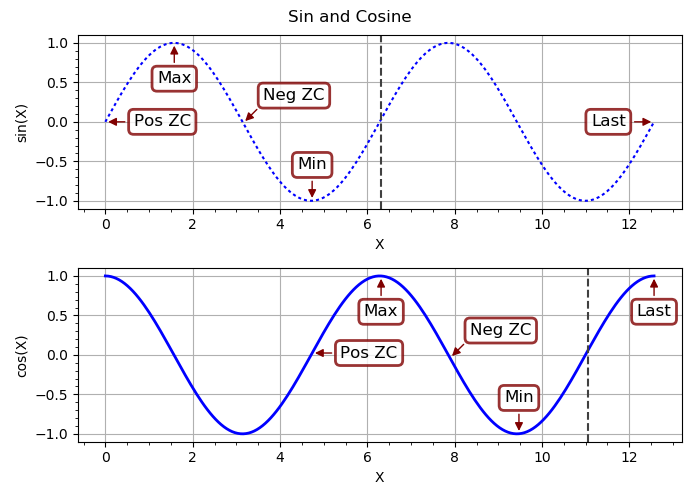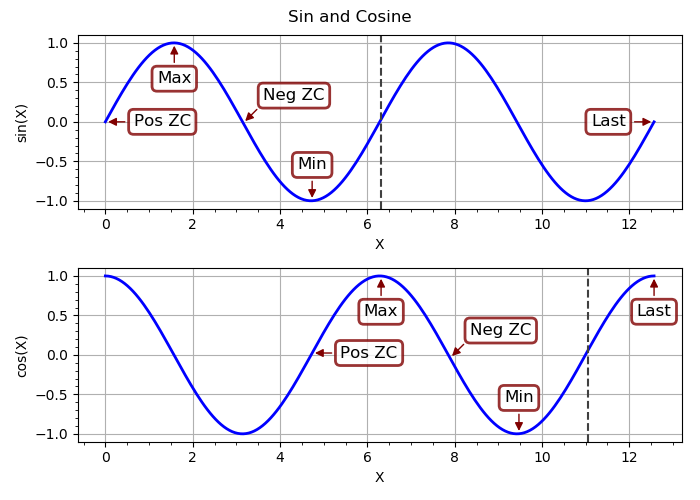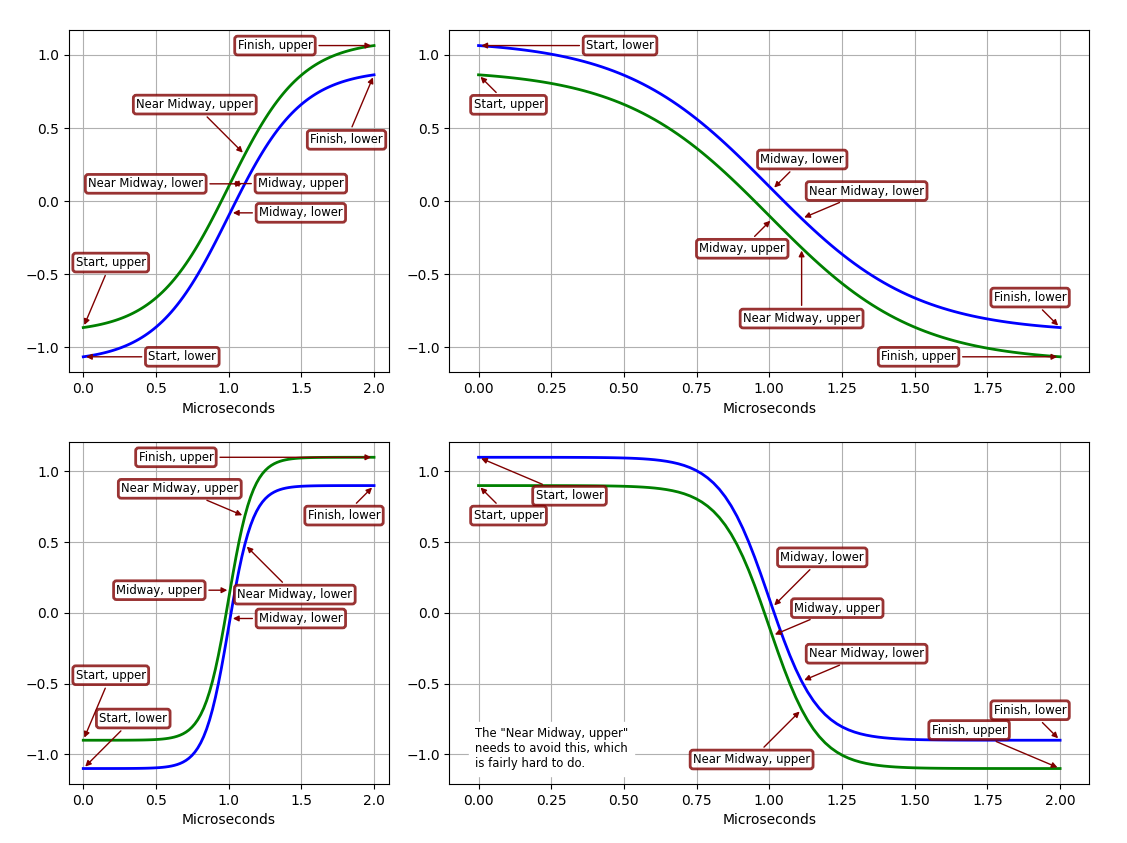# Software

## yampex

Yet Another Matplotlib Extension, with simplified subplotting

The Python 3 package yampex makes Matplotlib easier to use, especially with subplots and annotations. You simply construct a Plotter object with the number of subplot rows and columns you want, and do a context call on it to get a version of the object that’s all set up to do your subplots. Like this:

```import numpy as np
from yampex import Plotter

funcNames = ('sin', 'cos')
X = np.linspace(0, 4*np.pi, 200)
pt = Plotter(1, 2, width=7, height=5)
pt.set_title("Sin and Cosine")
pt.set_xlabel("X"); pt.set_grid()
with pt as p:
for funcName in funcNames:
if funcName == 'sin':
p.set_ylabel("{}(X)".format(funcName))
Y = getattr(np, funcName)(X)
p(X, Y)
pt.show()
```

The `Plotter` instance `pt` has various methods for setting plot modes. The example calls two of them before it makes the context call, thus affecting all subplots that are to be produced. It calls `pt.set_xlabel("X")` to make the x-axis label “X” and calls `pt.set_grid()` to include a grid, for all subplots.

Then the example code does a context call on the `pt` object to obtain a contextualized instance of it, `p`. Everything done to this contextualized instance applies to just one subplot rather than all subplots. So, when the example calls `p.set_ylabel` with a string-formatted argument, it sets the y-axis label of just one subplot at a time, based on the value of `funcName`.

The actual subplotting is done with a call to the `p` context object itself (not a method of it). The first argument is the x-axis vector `X`, and all arguments that follow are y-axis vectors plotted against the x-axis vector. In the example, there’s just one y-axis vector, `Y`. You can supply additional vectors to be plotted against the first x-axis one and they will show up in different colors.1

Once the `p` object has been called, the context switches to the next subplot. In the example, there are two subplots, one above the other. The second iteration of the `for` loop sets the second subplot’s y-axis label to “cos” and plots a cosine.

The end result of the example will look like this:Matplotlib subplotting, simplified

Notice that `p.add_line` was called for the first (top) subplot–to give it a dotted linestyle–but not for the second. The linestyle reverted to its solid default when the `p` object was called a second time for the bottom subplot. Each call to `p` inside the context gets a fresh start with the options that were set before (outside) the context.

### Annotations

Another thing that yampex can do for you is to annotate your plots with intelligently-positioned labels. You don’t have to worry about whether your annotation graphic will obscure part of the plot line, or sit on top of the plot borders looking ugly and weird. And adding the annotations is as simple as calling the `add_annotation` method of your plotter object. You make the call outside the with-as context if you want to add the annotation to all subplots, or in context to affect just one subplot.

Let’s expand the example to add annotations to the sine and cosine curves.

```import numpy as np
from yampex import Plotter

funcNames = ('sin', 'cos')
X = np.linspace(0, 4*np.pi, 200)
pt = Plotter(1, 2, width=7, height=5)
pt.set_title("Sin and Cosine")
pt.set_xlabel("X"); pt.set_grid()
with pt as p:
for funcName in funcNames:
Y = getattr(np, funcName)(X)
p.set_ylabel("{}(X)".format(funcName))
k = 0 if funcName == 'sin' else 75
for text in ("Pos ZC", "Max", "Neg ZC", "Min"):
k += 25
p.set_axvline(k)
p(X, Y)
pt.show()
```

First we add a global annotation to the overall Matplotlib-manager object `pt` that affects all subplots; the last point plotted is labeled “Last.” Then we enter the context manager and, via the contextualized object `p`, add annotations to each subplot for a positive zero crossing. The annotations are a maximum, a negative zero crossing, and a minimum. Here’s the result:Matplotlib with automatically placed annotation boxes!

Note how the annotation boxes are positioned so that they don’t cover up the plots or each other, or trespass on the borders. A surprisingly large amount of thought and computation went into making that happen. If you’re interested, you can check out the gory details of the `Annotator` class and its `Sizer`, `RectangleRegion`, and `PositionEvaluator` helpers. (This module is also, I submit, a fine example of the elegance and power of object-oriented programming.)

A couple of other things you might notice: There’s a dashed vertical line at the zero crossing after the last annotation. That was added by calling `p.set_axvline(k)`. Take a look at the methods of OptsBase to see all the options you can set. Another one that the example code has set (on a global scale, before entering the context manager) was the title for all subplots. You could just as easily set a different title for each subplot inside the context manager.

### A Complex Example

Here is a more complex example of an annotated plot, with four different-sized subplots. The curves and annotations were chosen to illustrate how yampex rises to the challenge of figuring out where to put the annotation text boxes without having them overlap either of the plotted lines, the subplot borders, or each other.A more complex subplot and annotation example

There’s a lot going on here. Click on the plot for a high-definition version and you can appreciate all the details. Imagine how your plots will look, and how much easier it will be to generate them in your own Python code! I wrote yampex for my own use, and will probably never produce Matplotlib plots without it.

### Running the Examples

After installing yampex with `pip install yampex`, you should have a new command added to your path, `yampex-examples`. You can just run that from the shell as a regular user and it will generate a subdirectory `~/yampex-examples` with some example files that can be run to generate plots. For example, you can generate the plot by typing `./annotate.py` in a shell from that subdirectory. Take a look at how small that file is. Here’s all the Python it contains:

```import numpy as np
from yampex import plot, annotate
from yampex.util import sub

class Figure(object):
verbose = False
multipliers = (1, 3)

def __init__(self):
# DEBUG: Set True to see positioning rectangles
if self.verbose:
annotate.Annotator.verbose = True
self.p = plot.Plotter(
2*len(self.multipliers),
verbose=self.verbose, width=1500, height=1000, w2=1)
self.p.use_timex()
self.p.use_grid()

for kVector in (0, 1):
text = sub("{}, {}", prefix, "upper" if kVector else "lower")

def plot(self):
def tb(text):

X = np.linspace(0, 2e-6, 100)
with self.p as self.sp:
for m in self.multipliers:
Y = np.tanh(m*2e6*(X-1e-6))
if self.sp.Nsp == 3:
tb("The \"Near Midway, upper\"")
tb("needs to avoid this, which")
tb("is fairly hard to do.")
self.sp(X, sign*(Y-0.1), sign*(Y+0.1))
self.p.show()

def run():
# Plot the curves
Figure().plot()

if __name__ == '__main__':
run()
```

That’s it!

You can modify any of these example files to learn how to use yampex, and your modifications will not be overwritten if you run `yampex-examples` again. To get the original example file, just delete the modified one and run `yampex-examples`, and the original will be copied.

The simplest example of all is to try out the package-level `xy` function. You can do that without installing any other examples. It provides ultra-simple XY plotting of a numerical sequence or Numpy vector, or a pair of Numpy vectors. Here is all you have to do in `ipython` or the Python console to try it:

```In : from yampex import xy
In : xy([0,1,2,1,0,-1,-2,-1,0])
```

With these two lines you get a sawtooth waveform. It’s a very handy little function when you’re trying to get a quick visualization of vectors in Python. If you want to plot to equal-length vectors against each other, just include them both as arguments.

Happy plotting!

Copyright (C) 2017-2021 by Edwin A. Suominen, http://edsuom.com/:

```See edsuom.com for API documentation as well as information about
Ed's background and other projects, software and otherwise.

you may not use this file except in compliance with the